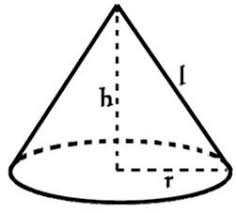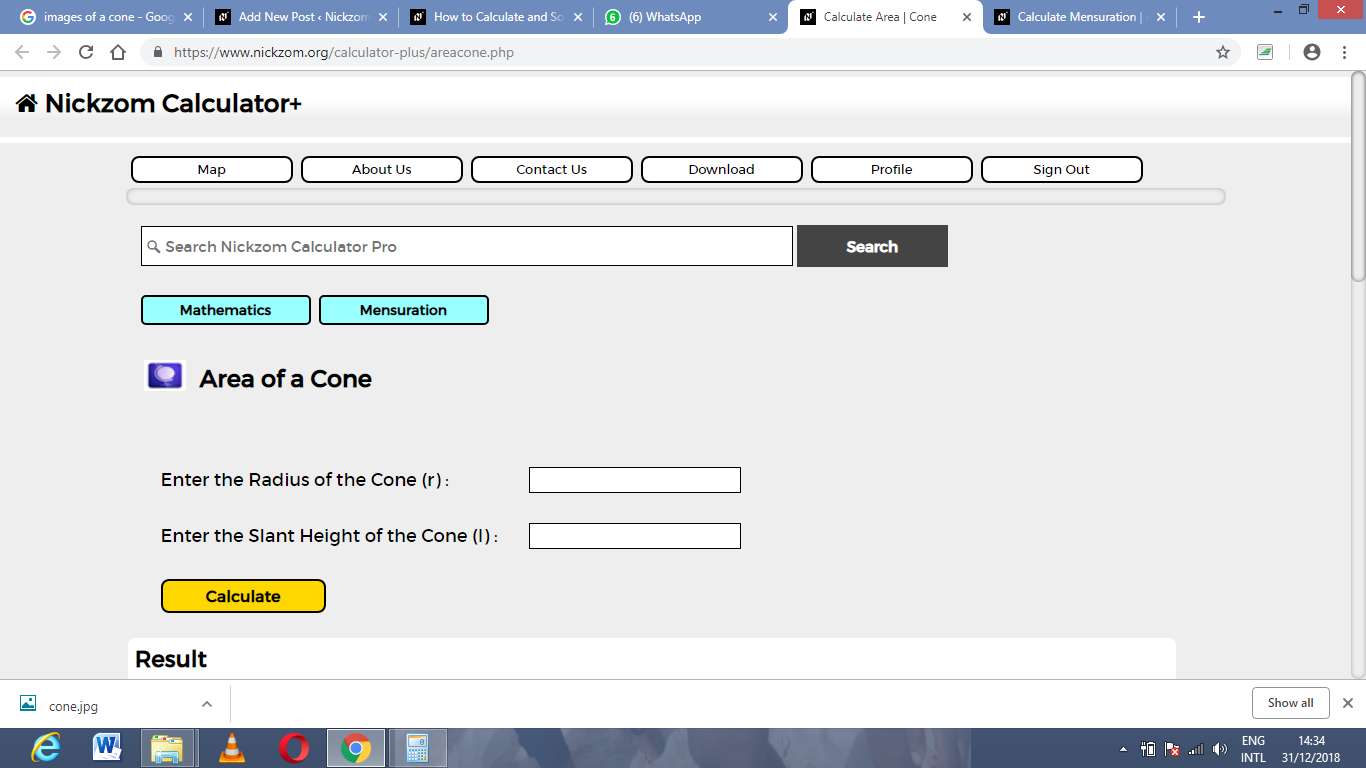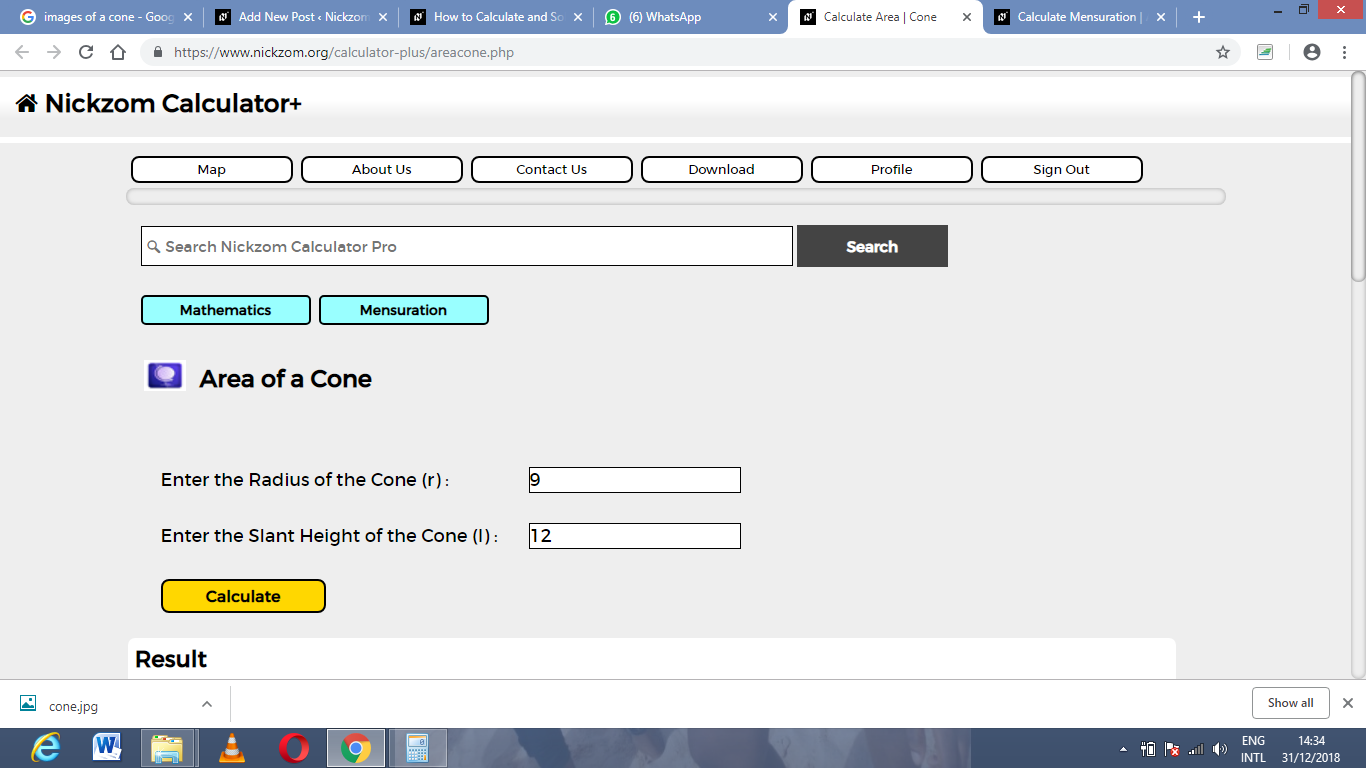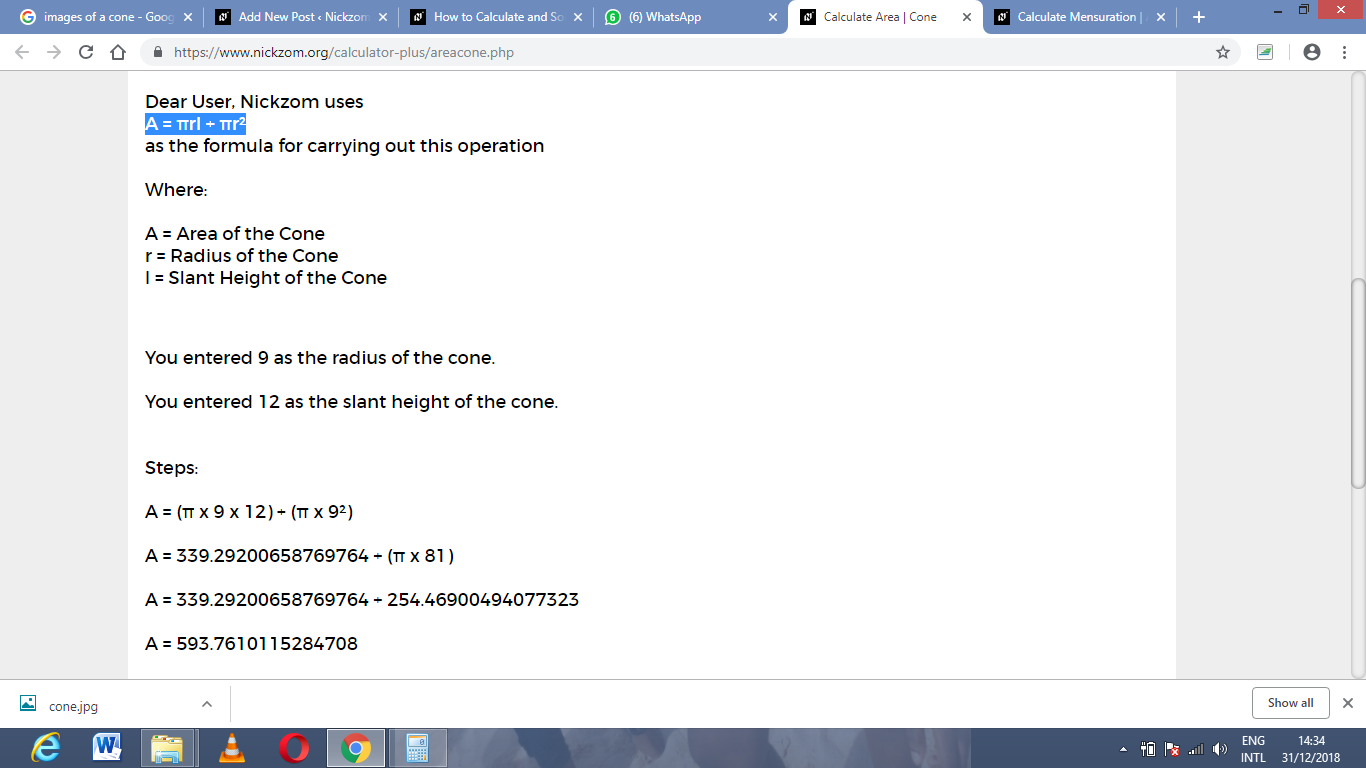# How to Calculate and Solve for the Area, Radius, Diameter and Slant Height of a Cone | The Calculator EncyclopediaThe image above is a cone.

To compute the area of a cone, two essential parameters is needed and this parameters are the radius of the cone (r) and the slant height of the cone (h).

The formula for calculating the area of a cone:

A = πrl + πr²

Where;

A = Area of the Cone
r = Radius of the Cone

Let’s solve an example:
Find the area of a cone when the radius of the cone is 9 cm and the slant height of the cone is 12 cm.

This implies that;
r = Radius of the cone = 9 cm
l = Slant height of the cone = 12 cm

A = πrl + πr²
A = 3.142 x 9 x 12 + 3.142 x 9²
A = 339.336 + 254.502
A =  593.83

Therefore, the area of the cone is 593.83 cm².

Calculating the Area of a cone using Diameter and Slant height of the cone.

A = πdl / 2 + πd2 / 4

Where;

d = Diameter of the Cone
l = Slant height of the Cone

Let’s solve an example:
Find the area of a cone when the diameter of the cone is 18 cm and the slant height of the cone is 22 cm?

This implies that;
d = diameter of the cone = 18 cm
l = Slant height of the cone = 22 cm

A = πdl / 2 + πd2 / 4
A = 3.142 x 18 x 22 / 2 + 3.142 (18)2 / 4
A = 1244.232 / 2 + 1018.008 / 4
A = 622.116 + 254.502
A = 876.6

Therefore, the area of the cone with diameter is 876.6 cm2.

Calculating the Slant height of a cone using Radius of the cone and Area of the cone.

l = A – πr2 / πr

Where;

A = Area of the Cone
r = Radius of the Cone

Let’s solve an example:
Find the slant height of a cone when the radius of the cone is 8 cm and the area of the cone is 220 cm2.

This implies that;
A = Area of the cone = 220 cm2
r = Radius of the cone = 8 cm

l = A – πr2 / πr
l = 220 – 3.142 x 82 / 3.142 x 8
l = 220 – 3.142 x 64 / 25.136
l = 220 – 201.088 / 25.136
l = 18.91 / 25.136
l = 0.75

Therefore, the slant height of the cone with radius is 0.75 cm.

Calculating the Slant height of a cone using Diameter of the cone and Area of the cone.

l = 4A – πd2 / 2πd

Where;

A = Area of the Cone
d = Diameter of the Cone

Let’s solve an example:
Find the slant height of a cone when the diameter of the cone is 16 cm and the area of the cone is 240 cm2.

This implies that;
A = Area of the cone = 240 cm2
d = Diameter of the cone = 16 cm

l = 4A – πd2 / 2πd
l = 4 x 240 – 3.142 x (16)2 / 2 x 3.142 x 16
l = 960 – 3.142 x 256 / 100.5
l = 960 – 804.35/ 100.5
l = 155.65 / 100.5
l = 1.548

Therefore, the slant height of the cone with diameter is 1.548 cm.

Nickzom Calculator – The Calculator Encyclopedia is capable of calculating the area of a cone.

To get the answer and workings of the area of a cone using the Nickzom Calculator – The Calculator Encyclopedia. First, you need to obtain the app.

You can get this app via any of these means:

To get access to the professional version via web, you need to register and subscribe for NGN 1,500 per annum to have utter access to all functionalities.
You can also try the demo version via https://www.nickzom.org/calculator

Once, you have obtained the calculator encyclopedia app, proceed to the Calculator Map, then click on Mensuration under the Mathematics sectionNow, click on Area of a Cone under MensurationThe screenshot below displays the page or activity to enter your values, to get the answer for the area of a cone according to the respective parameters which are the radius of the cone (r) and slant height of the cone (l)Now, enter the value appropriately and accordingly for the parameters as required by the example above where the radius of the cone is 9 cm and slant height of the cone is 12 cm.Finally, click on CalculateAs you can see from the screenshot above, Nickzom Calculator – The Calculator Encyclopedia solves for the area of a cone and presents the formula, workings and steps too.